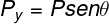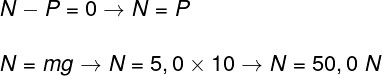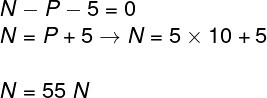# Normal force

Normal force is the reaction force that a surface exerts on any body that applies a force to it . When we walk, for example, we push against the ground. The ground, in turn, applies a normal force to our feet, which always acts in the perpendicular direction (90º angle) to the surface.

## normal force formula

The normal force does not have a specific formula , since it can vary according to the case studied. To calculate it, you need to apply Newton’s second law to each situation and determine what forces or components of forces act in each direction.

The simplest case involving the calculation of the normal force is one in which a body rests on a smooth, horizontal surface, in a region where there is gravity . In this case, the normal force is equal, in magnitude, to the weight of that body.

## Normal strength and weight strength

When an object rests on a horizontal surface, the force of weight , which pulls it down, causes that object to compress the surface, which “responds”, producing a reaction force on the object, called the normal force.

Although the normal force and the gravity force act in the same direction and have opposite directions, these two forces are not an action-reaction pair , simply because they act on the same body. According to Newton’s 3rd law , action and reaction forces always act on different bodies.

## Normal force on the inclined plane

The inclined plane is common in a wide range of situations and exercises. It consists of a straight plane that is rotated at an angle to the horizontal direction. On the inclined plane, the normal force is less than in the situation where the body is supported on a horizontal surface. This is because, in this case, the normal force cancels out only with the vertical component of the weight force.

In this case, the normal force is equivalent to the vertical component of the weight force , that is:P – weight force

Py – y component of the weight force

## Exercises solved on normal strength

Question 1 — A body of mass 5.0 kg is at rest and resting on a perfectly horizontal surface. Determine the magnitude of the normal reaction force acting on this body. Use g = 10 m/s².

a) 200 N

b) 100 N

c) 25 N

d) 50 N

Resolution :

Letter D.

As the body is at rest and, consequently, in the condition of balance of forces, the vertical resultant, given by the difference between the normal forces and weight, is zero. Therefore, the normal force has a magnitude equal to the weight of that body.Question 2 — A 5.0 kg object rests on an inclined plane that makes an angle of 60° with the horizontal direction. Determine the magnitude of the normal force that the inclined plane produces on such an object. Use g = 10 m/s².

a) 100 N

b) 12.5 N

c) 50 N

d) 25 N

Resolution:

Letter D.

The object resting on the inclined plane is acted upon by a force that is perpendicular to the surface of the inclined plane and which, therefore, corresponds to the vertical component of the weight force.Question 3 — A 5.0 kg object is resting on a flat, horizontal surface, so a force of 5 N is now applied to this block, in the vertical direction and pointing down. Determine the magnitude of the normal force acting on this block. Use g = 10 m/s².

a) 15 N

b) 25 N

c) 30 N

d) 55 N

Resolution:

Letter D.

In addition to the weight force, there is a force of 5 N acting downwards, yet the block remains at rest. Therefore, the resultant of the normal forces—which point up—, weight, and the 5N force—which point down—is zero.Check Also
Close